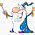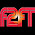## Monday, June 23, 2014

### 33 | Katie Couric's East Hampton Marriage

Katie Couric married John Molner today, someone with name numerology just like John Kerry.  The wedding took place at Katie Couric's home in East Hampton.  She lives in a big house because she tells big lies- that's how it works.
• Katie = 2+1+2+9+5 = 19
• Couric = 3+6+3+9+9+3 = 33
• John = 10+15+8+14 = 47
• Molner = 13+15+12+14+5+18 = 77
Like many elites with "33" names, she also lives in a city with a "33" name.
• Hampton 8+1+4+7+2+6+5 = 33
People magazine was their to photograph the wedding.
• People = 7+5+6+7+3+5 = 33
Couric is 57 and Molner is 51.
• Katie Couric-Molner = 2+1+2+9+5+3+6+3+9+9+3+4+6+3+5+5+9 = 84

1.2.